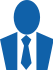# MOR toolbox

MOR is a MATLAB® based toolbox gathering algorithms for

• reduction of large-scale linear dynamical models,
• creation of linear dynamical models from input-output frequency data.

The algorithms gathered in the MOR toolbox generate a linear state-space model which input-output behaviour is close to the initial model. Several tools for analysis are also provided to evaluate the relevance of the reduced-order model.

Several methods and options are available, allowing for a fine tuning of the approximation process to suit your needs. For instance, one can perform an approximation over a bounded frequency interval, preserve the stability, the static gain or some eigenvalues. Some methods take advantage of the sparse nature of the models and can therefore be applied to very large-scale models with several thousands of states. Such models arise very often in physics, biology, etc.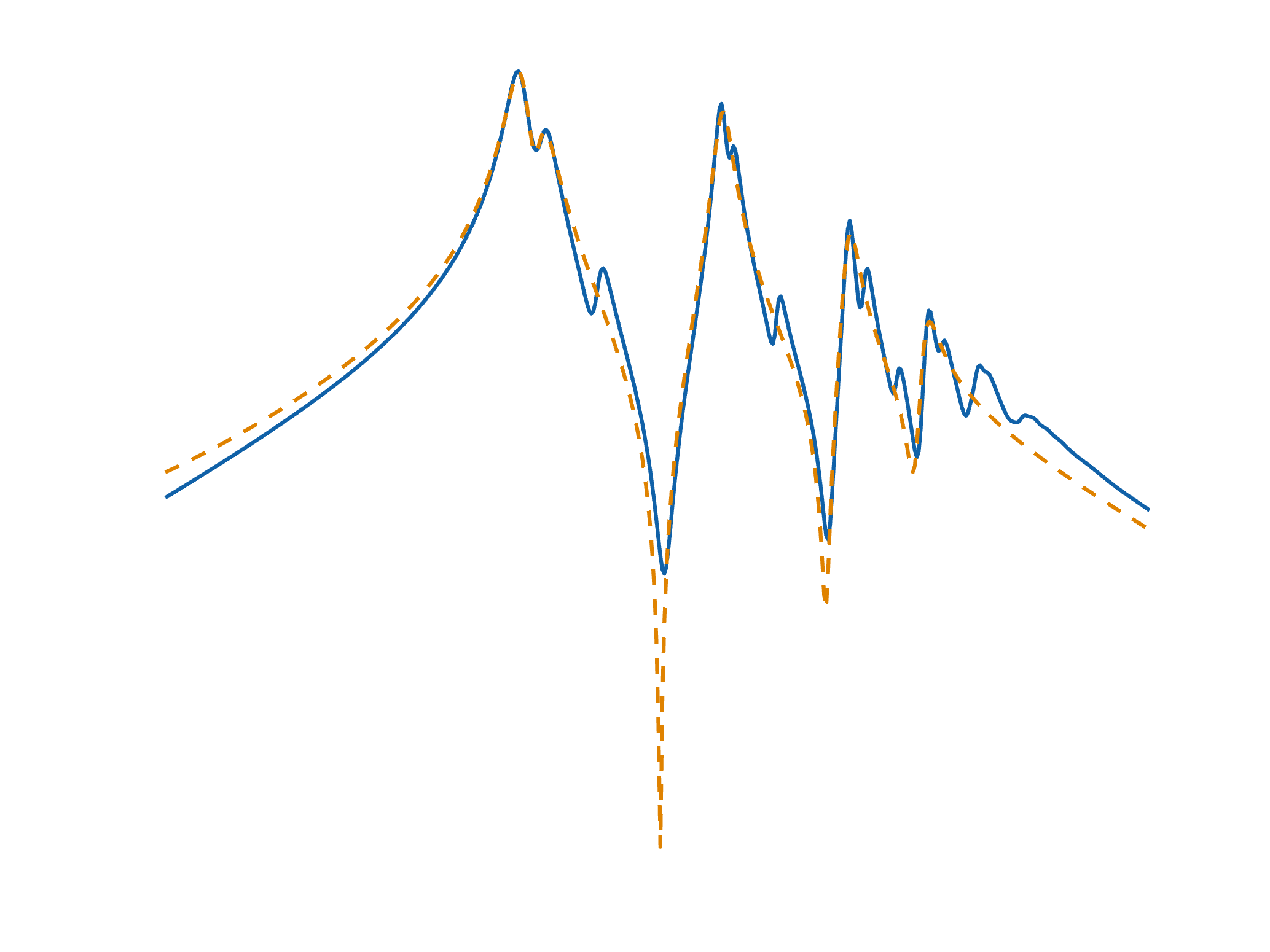# MOR toolbox

Reduction of linear dynamical models

# Features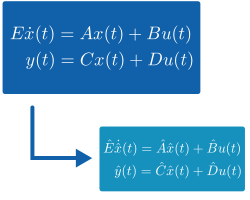# Reduction of linear model given as state-space

Obtain a reduced-order model from a large-scale dynamical model described by a state-space representation.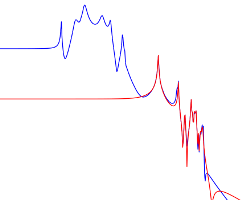# Reduction over a bounded frequency interval

Obtain a reduced-order model that is very accurate in a specific frequency interval of interest.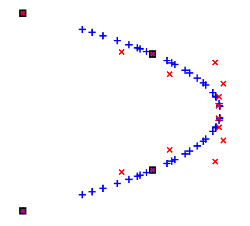# Preserve some user-defined eigenvalues

Obtain a reduced-order model that preserves some of the eigenvalues of the initial model to retrieve some physical properties.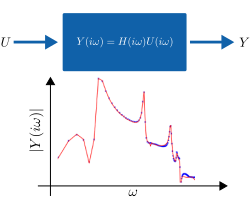# Approximation from data obtained with a frequency-domain simulator

Obtain a dynamical model of appropriate complexity from data collected with a frequency-domain simulator of your system.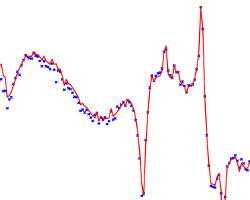# Approximation from experimental data

Obtain a dynamical model from experimental data, collected during real experiments.

# Try it or buy itThe MOR toolbox is free for an academic use.